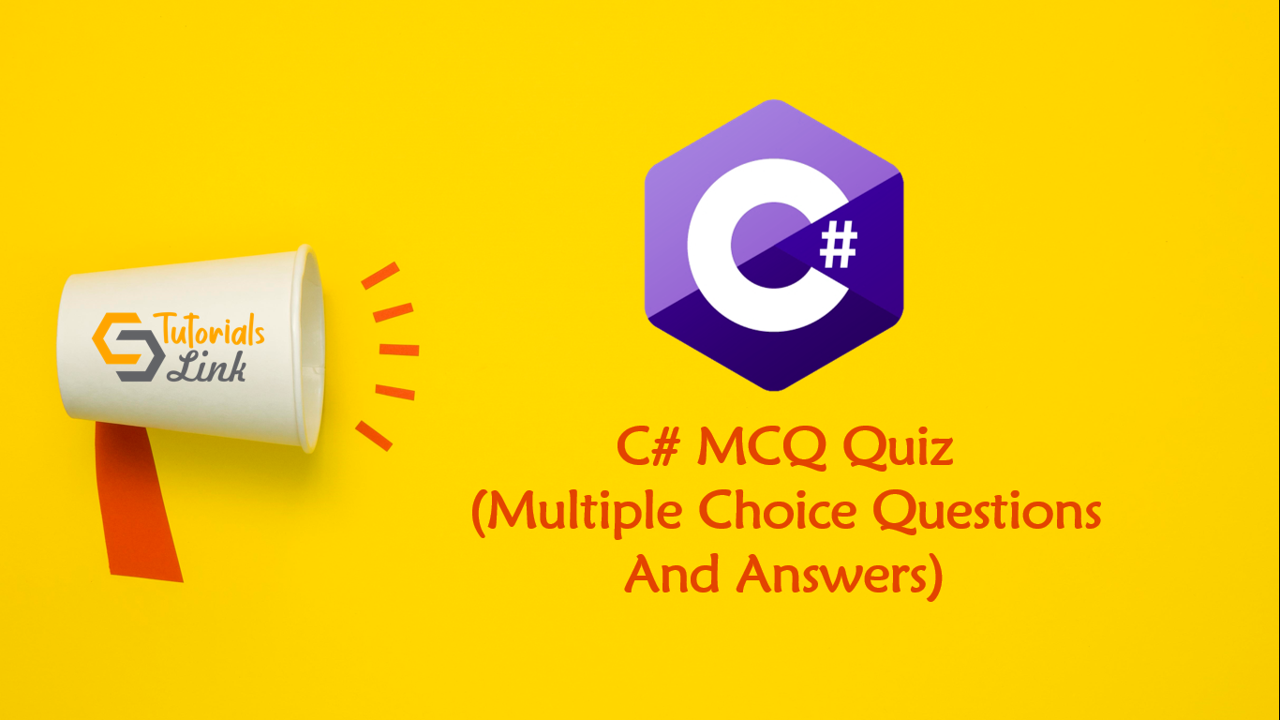Loading, please wait...# C# Multiple Choice Questions And Answers

Search here for MCQs

Are you preparing for the next job interviews? If yes, trust me this post will help you also we'll suggest you check out a big collection for Programming Full Forms that may help you in your interview:

## 11. Select the two types of threads mentioned in the concept of multithreading?

• Foreground
• Background
• Only foreground
• Both foreground and background

## 12. Choose the wrong statement about properties used in C#.Net?

• Each property consists of accessor as getting and set.
• A property cannot be either read or write-only.
• Properties can be used to store and retrieve values to and from the data members of a class.
• Properties are like actual methods that work like data members.

## 13. If a class ‘demo’ had ‘add’ property with getting and set accessors, then which of the following statements will work correctly?

• math m =  new math(); m.add = 10;
• None of the mentioned

## 14. What will be the output of the following code snippet?

``````using System;
class sample
{
int i;
double k;
public sample (int ii, double kk)
{
i = ii;
k = kk;
double j = (i) + (k);
Console.WriteLine(j);
}
~sample()
{
double j = i - k;
Console.WriteLine(j);
}
}
class Program
{
static void Main(string[] args)
{
sample s = new sample(9, 2.5);
}
}``````
• 0   0
• 11.5   0
• Compile-time error
• 11.5 6.5

## 15. What will be the output of the following code snippet?

``````using System;

class program
{
static void Main(string[] args)
{
int x = 8;
int b = 16;
int c = 64;
x /= c /= b;
Console.WriteLine(x + " " + b+ " " +c);